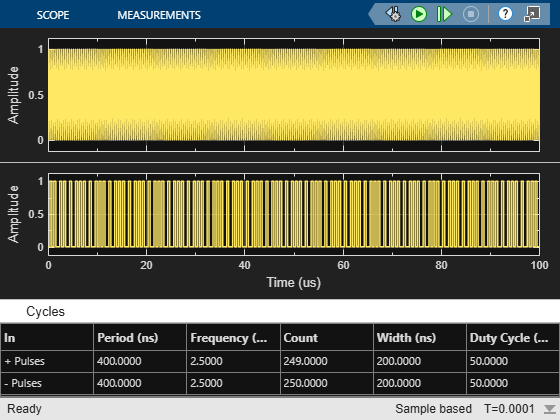# Frequency Division Using Fractional Clock Divider with DSM

Open the model `fractionalClockDivider_w_DSM`. The model consists of a Pulse Generator and a Fractional Clock Divider with Accumulator block.

```model='fractionalClockDivider_w_DSM'; open_system(model) ```The period of the incoming pulse at the clk in port is `4e-7` s. So, the incoming signal has a frequency of `2.5` MHz. The div-by value is set at `2.5`. The clock divider uses a second order delta sigma modulator.

Run the simulation for `1e-4` s. The frequency of the output signal is `1.002` MHz.

```sim(model); ```